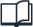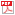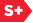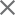Sample Pages### Spie Press Book

Optical Imaging and Aberrations, Part III: Wavefront Analysis
Author(s): Virendra N. Mahajan
Format Member Price Non-Member Price

Book Description

Wavefront Analysis is Part III of a series of books on Optical Imaging and Aberrations. It has evolved out of the author's work and lectures over the years on wavefront analysis as applied to optical design and testing. Its focus is on the use of orthonormal polynomials that represent balanced classical aberrations in optical imaging systems with pupils of various shapes. After a brief introduction to optical imaging, aberrations, and orthonormalization of a set of polynomials over a certain domain to obtain polynomials that are orthonormal over another domain, this book describes in detail the polynomials appropriate for various shapes of the system pupil. Starting with the system that is most common in imaging, namely, the one with a circular pupil, systems with annular, hexagonal, elliptical, rectangular, square, and slit pupils are considered. Included in this list are also systems with circular and annular pupils with Gaussian illumination, anamorphic systems with square and circular pupils, and those with circular and annular sector pupils. These chapters start with a brief discussion of aberration-free imaging that includes both the PSF and the OTF of a system. A separate chapter is devoted to a discussion of the pitfalls of using the Zernike circle polynomials for systems with noncircular pupils by applying them to systems with annular and hexagonal pupils. Similarly, a chapter is devoted to the calculation of orthonormal aberration coefficients from the wavefront or the wavefront slope data. Each chapter ends with a brief summary that describes the essence of its content.

Book Details

Date Published: 7 August 2013
Pages: 448
ISBN: 9780819491114
Volume: PM221
Errata

Preface
Acknowledgments
Symbols and Notation

1. OPTICAL IMAGING
1.1 Introduction
1.2 Diffraction Image
1.2.1 Pupil Function
1.2.2 PSF
1.2.3 OTF
1.3 Strehl Ratio
1.3.1 General Expression
1.3.2 Approximate Expression in Terms of Aberration Variance
1.4 Aberration Balancing
1.5 Summary
References

2. OPTICAL WAVEFRONTS AND THEIR ABERRATIONS
2.1 Introduction
2.2 Optical Imaging
2.3 Wave and Ray Aberrations
2.4 Defocus Aberration
2.5 Wavefront Tilt
2.6 Aberration Function of a Rotationally Symmetric System
2.7 Observation of Aberrations: Interferograms
2.8 Summary
References

3. ORTHONORMAL POLYNOMIALS AND GRAM-SCHMIDT ORTHONORMALIZATION
3.1 Introduction
3.2 Orthonormal Polynomials
3.3 Equivalence of Orthogonality-Based Coefficients and Least-Squares Fitting
3.4 Orthonormalization of Zernike Circle Polynomials over Noncircular Pupils
3.5 Unit Pupil
3.6 Summary
References

4. SYSTEMS WITH CIRCULAR PUPILS
4.1 Introduction
4.2 Pupil Function
4.3 Aberration-Free Imaging
4.3.1 PSF
4.3.2 OTF
4.4 Strehl Ratio and Aberration Tolerance
4.4.1 Strehl Ratio
4.4.2 Defocus Strehl Ratio
4.4.3 Approximate Expressions for Strehl Ratio
4.5 Balanced Aberrations
4.6 Description of Zernike Circle Polynomials
4.6.1 Analytical Form
4.6.2 Circle Polynomials in Polar Coordinates
4.6.3 Polynomial Ordering
4.6.4 Number of Circle Polynomials through a Certain Order n
4.6.5 Relationships among the Indices n, m, and j
4.6.6 Uniqueness of Circle Polynomials
4.6.7 Circle Polynomials in Cartesian Coordinates
4.7 Zernike Circle Coefficients of a Circular Aberration Function
4.8 Symmetry Properties of Images Aberrated by a Circle Polynomial Aberration
4.8.1 Symmetry of PSF
4.8.2 Symmetry of OTF
4.9 Isometric, Interferometric, and Imaging Characteristics of Circle Polynomial Aberrations
4.9.1 Isometric Characteristics
4.9.2 Interferometric Characteristics
4.9.3 PSF Characteristics
4.9.4 OTF Characteristics
4.10 Circle Polynomials and Their Relationships with Classical Aberrations
4.11 Zernike Coefficients of a Scaled Pupil
4.11.1 Theory
4.11.2 Application to a Seidel Aberration Function
4.11.3 Numerical Example
4.12 Summary
References

5. SYSTEMS WITH ANNULAR PUPILS
5.1 Introduction
5.2 Aberration-Free Imaging
5.2.1 PSF
5.2.2 OTF
5.3 Strehl Ratio and Aberration Balancing
5.4 Orthonormalization of Circle Polynomials over an Annulus
5.5 Annular Polynomials
5.6 Annular Coefficients of an Annular Aberration Function
5.7 Strehl Ratio for Annular Polynomial Aberrations
5.8 Isometric, Interferometric, and Imaging Characteristics of Annular Polynomial Aberrations
5.9 Summary
References

6. SYSTEMS WITH GAUSSIAN PUPILS
6.1 Introduction
6.2 Gaussian Pupil
6.3 Aberration-Free Imaging
6.3.1 PSF
6.3.3 OTF
6.4 Strehl Ratio and Aberration Balancing
6.5 Orthonormalization of Zernike Circle Polynomials over a Gaussian Circular Pupil
6.6 Gaussian Circle Polynomials Representing Balanced Primary Aberrations for a Gaussian Circular Pupil
6.7 Weakly Truncated Gaussian Pupils
6.8 Aberration Coefficients of a Gaussian Circular Aberration Function
6.9 Orthonormalization of Annular Polynomials over a Gaussian Annular Pupil
6.10 Gaussian Annular Polynomials Representing Balanced Primary Aberrations for a Gaussian Annular Pupil
6.11 Aberration Coefficients of a Gaussian Annular Aberration Function
6.12 Summary
References

7. SYSTEMS WITH HEXAGONAL PUPILS
7.1 Introduction
7.2 Pupil Function
7.3 Aberration-Free Imaging
7.3.1 PSF
7.3.2 OTF
7.4 Hexagonal Polynomials
7.5 Hexagonal Coefficients of a Hexagonal Aberration Function
7.6 Isometric, Interferometric, and Imaging Characteristics of Hexagonal Polynomial Aberrations
7.7 Seidel Aberrations, Standard Deviation, and Strehl Ratio
7.7.1 Defocus
7.7.2 Astigmatism
7.7.3 Coma
7.7.4 Spherical Aberration
7.7.5 Strehl Ratio
7.8 Summary
References

8. SYSTEMS WITH ELLIPTICAL PUPILS
8.1 Introduction
8.2 Pupil Function
8.3 Aberration-Free Imaging
8.3.1 PSF
8.3.2 OTF
8.4 Elliptical Polynomials
8.5 Elliptical Coefficients of an Elliptical Aberration Function
8.6 Isometric, Interferometric, and Imaging Characteristics of Elliptical Polynomial Aberrations
8.7 Seidel Aberrations and Their Standard Deviations
8.7.1 Defocus
8.7.2 Astigmatism
8.7.3 Coma
8.7.4 Spherical Aberration
8.8 Summary
References

9. SYSTEMS WITH RECTANGULAR PUPILS
9.1 Introduction
9.2 Pupil Function
9.3 Aberration-Free Imaging
9.3.1 PSF
9.3.2 OTF
9.4 Rectangular Polynomials
9.5 Rectangular Coefficients of a Rectangular Aberration Function
9.6 Isometric, Interferometric, and Imaging Characteristics of Rectangular Polynomial Aberrations
9.7 Seidel Aberrations and Their Standard Deviations
9.7.1 Defocus
9.7.2 Astigmatism
9.7.3 Coma
9.7.4 Spherical Aberration
9.8 Summary
References

10. SYSTEMS WITH SQUARE PUPILS
10.1 Introduction
10.2 Pupil Function
10.3 Aberration-Free Imaging
10.3.1 PSF
10.3.2 OTF
10.4 Square Polynomials
10.5 Square Coefficients of a Square Aberration Function
10.6 Isometric, Interferometric, and Imaging Characteristics of Square Polynomial Aberrations
10.7 Seidel Aberrations and Their Standard Deviations
10.7.1 Defocus
10.7.2 Astigmatism
10.7.3 Coma
10.7.4 Spherical Aberration
10.8 Summary
References

11. SYSTEMS WITH SLIT PUPILS
11.1 Introduction
11.2 Aberration-Free Imaging
11.2.1 PSF
11.2.2 Image of an Incoherent Slit
11.3 Strehl Ratio and Aberration Balancing
11.3.1 Strehl Ratio
11.3.2 Aberration Balancing
11.4 Slit Polynomials
11.5 Standard Deviation of a Primary Aberration
11.3 Summary
References

12. USE OF ZERNIKE CIRCLE POLYNOMIALS FOR NONCIRCULAR PUPILS
12.1 Introduction
12.2 Relationship Between the Orthonormal and the Corresponding Zernike Circle Coefficients
12.3 Use of Zernike Circle Polynomials for the Analysis of an Annular Wavefront
12.3.1 Zernike Circle Coefficients in Terms of the Annular Coefficients
12.3.2 Interferometer Setting Errors
12.3.3 Wavefront Fitting
12.3.4 Application to an Annular Seidel Aberration Function
12.4 Use of Zernike Circle Polynomials for the Analysis of a Hexagonal Wavefront
12.4.1 Zernike Circle Coefficients in Terms of Hexagonal Coefficients
12.4.2 Interferometer Setting Errors
12.4.3 Numerical Example
12.5 Aberration Coefficients from Discrete Wavefront Data
12.6 Summary
References

13. ANAMORPHIC SYSTEMS
13.1 Introduction
13.2 Gaussian Imaging
13.3 Classical Aberrations
13.4 Strehl Ratio and Aberration Balancing for a Rectangular Pupil
13.5 Aberration Polynomials Orthonormal over a Rectangular Pupil
13.6 Expansion of a Rectangular Aberration Function in Terms of Orthonormal Rectangular Polynomials
13.7 Anamorphic Imaging System with a Circular Pupil
13.7.1 Balanced Aberrations
13.7.2 Orthonormal Polynomials Representing Balanced Aberrations
13.8 Comparison of Polynomials for Rotationally Symmetric and Anamorphic Imaging Systems
13.9 Summary
References

14. NUMERICAL WAVEFRONT ANALYSIS
14.1 Introduction
14.2 Zernike Coefficients from Wavefront Data
14.2.1 Theory
14.2.2 Numerical Example
14.3 Zernike Coefficients from Wavefront Slope Data
14.3.1 Theory
14.3.2 Alternative Approach for Obtaining Zernike Coefficients from Wavefront Slope Data
14.3.3 Numerical Example
14.4 Summary
References

APPENDIX: SYSTEMS WITH SECTOR PUPILS

### Preface

This book is Part III of a series of books on Optical Imaging and Aberrations. Part I on Ray Geometrical Optics and Part II on Wave Diffraction Optics were published earlier. Part III is on Wavefront Analysis, which is an integral part of optical design, fabrication, and testing. In optical design, rays are traced to determine the wavefront and thereby the quality of a design. In optical testing, the fabrication errors and, therefore, the associated aberrations are measured by way of interferometry. In both cases, the quality of the wavefront is determined from the aberrations obtained at an array of points. The aberrations thus obtained are used to calculate the mean, the peak-to-valley, and the standard deviation values. While such statistical measures of the wavefront are part of wavefront analysis, the purpose of this book is to determine the content of the wavefront by decomposing the ray-traced or test-measured data in terms of polynomials that are orthogonal over the expected domain of the data. These polynomials must include the basic aberrations of wavefront defocus and tilt, and represent balanced classical aberrations.

We start Part III with an outline of optical imaging in the presence of aberrations in Chapter 1, i.e., on how to obtain the point-spread and optical transfer functions of an imaging system with an arbitrary shaped pupil. The Strehl ratio of a system as a measure of image quality is introduced in this chapter, and shown to be dependent only on the aberration variance when the aberration is small. It is followed in Chapter 2 with a brief discussion of the wavefronts and aberrations. This chapter introduces the nomenclature of aberrations. How to obtain the orthogonal polynomials over a certain domain from those over another is discussed in Chapter 3. For systems with a circular pupil, the Zernike circle polynomials are well known for wavefront analysis. They are discussed at length in Chapter 4. These polynomials are orthogonalized over an annular pupil in Chapter 5, and over a Gaussian pupil in Chapter 6. They are obtained similarly for systems with hexagonal, elliptical, rectangular, square, and slit pupils in the succeeding chapters. For each pupil, the polynomials are given in their orthonormal form so that an expansion coefficient (with the exception of piston) represents the standard deviation of the corresponding polynomial aberration term. The standard deviation of a Seidel aberration with and without aberration balancing is also discussed in these chapters.

Since the Zernike circle polynomials form a complete set, a wavefront over any domain can be expanded in terms of them. However, the pitfalls of their use over a domain other than circular and resulting from the lack of their orthogonality over the chosen domain are discussed in Chapter 12. Finally, the aberrations of anamorphic systems are discussed, and polynomials suitable for their aberration analysis are given in Chapter 13 for both rectangular and circular pupils. The use of the orthonormal polyonomials for determining the content of a wavefront is demonstrated in Chapter 14 by computer simulations of circular wavefronts. The determination of the aberrations coefficients from the wavefront slope data, as in a Shack-Hartmann sensor, is also discussed in this chapter.

Virendra N. Mahajan
El Segundo, California
June 2013